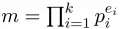## Day 2, Problem 3 - Robot No. M

It is the year 3030, and Macsy is currently producing a batch of robots on Mars.

During the 1st second, he transfers robot no. 1 onto Mars. Robot no. 1 is used to produce more robots.
During the 2nd second, robot no. 1 produces its first robot — robot no. 2.
During the 3rd second, robot no. 1 produces another robot — robot no. 3.
Each second hereafter, robot no. 1 produces a new robot. The robot produced during the m-th second is known as robot no. m.

After a robot is produced, it immediately begins work. Robot no. m will rest once every m seconds. For example, robot no. 3 will rest during the 6th, 9th, 12th, … seconds, working during all other times.

When a robot is at rest, its memory will be ported to the robot born at that second. For example, when robot no. 6 is born, robots no. 2 and 3 are resting. Thus, robot no. 6 will receive a copy of the memories of robots no. 2 and 3. We shall call robots no. 2 and 3 the teachers of robot no. 6.

If two robots are not teacher and student, and also do not share the same teacher, then the knowledge of these two robots are considered mutually independent. Note: Robot no. 1's knowledge is independent with respect to all other robots (since only robot no. 1 can produce other robots), it is also not any other robot's teacher.

A robot's independency number refers to the number of robots numbered small than it, while also being independent to it. For example, robot no. 1 has an independency number of 0, robot no. 2 has an independency number of 1 (robot no. 1 is independent to it), and robot no. 6 has an independency number of 2 (robots no. 1 and 5 are independent to it, robots no. 2 and 3 are both its teachers, and robot no. 4 share the same teacher with it — robot no. 2).

The newly produced robots have 3 different types of jobs. For a given robot no. m, if one can express m as the product of an even number of distinct, odd primes, then the robot is a politician (e.g. robot no. 15). Otherwise, if m is already an odd prime, or m can be expressed as the product of an odd number of distinct, odd primes, then the robot is a soldier (e.g. robots no. 3 and 165). All other robots are scholars, such as robots no. 2, 6, and 9.

Robot no. m born during the m-th second would like to know, amongst all of its teachers and itself, the sum of the independency scores of all the politicians, the sum of the independency scores of all the soldiers, and the sum of the independency scores of all the scholars. However, robot no. m is too busy with work and has no spare time to calculate this. Can you help it?

To simplify your calculations, Macsy has already performed a factorization of m. To simplify the output, you are only required to find the sums modulo 10000.

### Input Format

The first line of input contains the positive integer k (1 ≤ k ≤ 1000), the number of distinct prime factors of m.
The next k lines will each contain two integers pi and ei, representing the i-th distinct prime factor and its exponent (i = 1, 2, …, k). If p1, p2, …, pk are the distinct prime factors, then$\textstyle m=\prod_{i=1}^{k}p_i^{e_i}$. All the factors will be given in increasing order p1 < p2 < … < pk. In the input, the constraints 2 ≤ pi < 10,000 and 1 ≤ ei ≤ 1,000,000 will hold.

### Output Format

The output should contain three lines.
Line 1 should contain the sum of the independency scores of all the politicians amongst robot no. m and all of its teachers, modulo 10000.
Line 2 should contain the sum of the independency scores of all the soldiers amongst robot no. m and all of its teachers, modulo 10000.
Line 2 should contain the sum of the independency scores of all the scholars amongst robot no. m and all of its teachers, modulo 10000.

```3
2 1
3 2
5 1
```

```8
6
75
```

### Explanation

m = 2×32×5 = 90. Robot no. 90 has 10 teachers, yielding a total of 11 robots counting itself. Out of these robots, only robot no. 15 is a politician. The soldiers are robots no. 3 and 5. There are 8 scholars, and their numbers are respectively 2, 6, 9, 10, 18, 30, 45, and 90.

### Scoring

The output contains 3 numbers. For each test case, your score out of 10 will be:

• 10 if your program calculates all 3 numbers correctly;
• 7 if your program only calculates 2 numbers correctly;
• 4 if your program only calculates 1 number correctly; and
• 0 if your program does not calculate any number correctly.

Point Value: 25 (partial)
Time Limit: 2.00s
Memory Limit: 16M